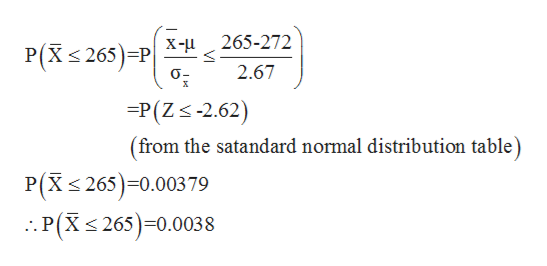# Suppose the lengths of the pregnancies of a certain animal are approximately normally distributed with mean μ=272 days and standard deviation σ=19 days.What is the probability that a random sample of 51 pregnancies has a mean gestation period of 265 days or​ less?

Question
Suppose the lengths of the pregnancies of a certain animal are approximately normally distributed with mean μ=272 days and standard deviation σ=19 days.

What is the probability that a random sample of 51 pregnancies has a mean gestation period of 265 days or​ less?

check_circle

Step 1

From the given information,

Mean (μ) =272days and standard deviation (σ) =19days

Sample size (n) =51

Step 2

Let ‘X’ be a normal distribution of a random variable

Now, standard deviation for sample mean is

Step 3

Now, probability for a mean gestatio...help_outlineImage Transcriptionclose265-272 х-и P(X 265)-P 2.67 х =P(Z-2.62) (from the satandard normal distribution table) P(X2650.00379 2. P(X 265 0.0038 fullscreen

### Want to see the full answer?

See Solution

#### Want to see this answer and more?

Solutions are written by subject experts who are available 24/7. Questions are typically answered within 1 hour.*

See Solution
*Response times may vary by subject and question.
Tagged in

### Statistics